##### Page tree

MagicDraw 19.0 LTR SP3 Documentation

Once you have created the Metric Table, which represents the metrics calculated at the time you created, you can make further calculations and track the evolution of your model development.
If you need to append new metrics to your table, you can easily open it.

### Opening the Metric Table

To open the Metric Table

1. In the Containment tree, right-click the element whose Metric Table you need to append.
2. On the shortcut menu of the element, click Tools > Metrics and then select the Metric Table you need to append.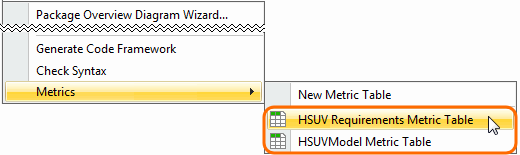The selected Metric Table opens. Now you are ready to calculate new metrics.

### Calculating new metrics

To calculate new metrics

1. In the Metric Table, select the row. Below that, you need to add a row with newly calculated metrics.
2. Do one of the following:
• On the Edit toolbar, click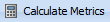and then select Calculate New Metric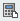from the open menu.
• Right-click the selected row and then click Calculate New Metricon the shortcut menu.

The new metrics are calculated and represented in the table below the selected row.

Excluding auxiliary resources from validation-based metrics calculation

By default, validation-based metrics are calculated in used read-only projects (auxiliary resources) as well. To exclude auxiliary resources from metric calculation scope, click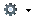in the Metric Table toolbar and select the Exclude Elements from Used Read-Only Projects option.

### Recalculating metrics

To recalculate metrics

1. In the Metric Table, select the row whose metrics you need to recalculate.
2. Do one of the following:
• On the Edit toolbar, clickand then select Recalculate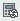from the open menu.
• Right-click the selected row and then click Recalculateon the shortcut menu.

The new metrics are calculated and represented in the table, instead of the selected row.

### Calculating new metrics with different parameter values

To calculate new metrics with different parameter values

1. In the Metric Table, select the row. Below that, you need to add a row for representing newly calculated metrics.
2. Do one of the following:
• On the Edit toolbar, clickand then select Add New Metric with Different Parameters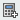from the open menu.
• Right-click the selected row and then click Add New Metric with Different Parameterson the shortcut menu.
3. A new row with copied parameters is added to the Metric table.

If the new row is empty, it might be that you haven’t selected any row. You may continue the procedure.

4. Change parameter values.
5. Select the newly created row and do one of the following:

• On the Edit toolbar, clickand then select Recalculatefrom the open menu.

• Right-click the row and then select Recalculatefrom the shortcut menu.

The new metrics are calculated according to the specified parameter values.

• No labels